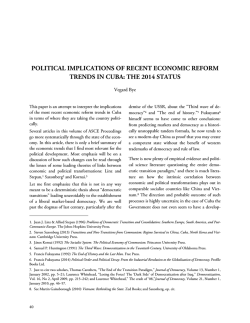# Jorge Perez-Lopez - Association for the Study of the Cuban Economy

```A SIMPLE GROWTH MODEL OF THE CUBAN ECONOMY
Carlos Seiglie
The objective of this paper is to analyze certain stylized facts of the Cuban economy. The first is the recent policy by the Cuban government to allow the
development of a private sector alongside the state
sector. The second is the push towards greater industrialization with the development of the port of
Mariel. The third is to explore the effects of a decline
in fertility in Cuba on its prospects for economic development. Finally, it appears that Cuba's model will
consist of closing inefficient state companies and permitting the existence of only a few to remain in order
to encourage competition between them and therefore efficiency.
The literature on the determinants of economic
growth in centrally planned economies is sparse. Notable contributions to the literature include Roberts
and Rodriguez (1997) and Bajona and Locay (2009).
This paper differs from theirs in that it models economic growth within a two sector model of capital
accumulation. It follows in the vein of Uzawa (1962,
1964) of a Solow-Swan type model for the sake of
simplicity.
THE MODEL
We assume two sectors in the economy, a private sector which we denote with subscript p and the state
sector which we denote by s. In this economy, labor
and capital are assumed to be freely mobile between
sectors. The production functions in both sectors are
assumed to exhibit constant returns to scale. The key
difference between this model and other two-sector
models is that competition is assumed to exist in the
private sector, but the state sector is a monopoly. In
other words, we assume that the Cuban government
does not permit entry into the state sector but does
allow entry and exit from the private sector. This is
in fact currently the case since the Cuban government has licensing requirement for entry into the
private sector, while not allowing entry into the state
sector. It should be noted that this policy of restricting entry into the state sectors will have consequences
for Cuba's trade policy in the sense that the Cuban
government will have to restrict international trade
(imports) in order to maintain the monopoly position of the state sector. Without the Cuban government restricting trade, imports of goods which compete directly with state produced goods will reduce
the profitability of government industries, and therefore, government revenues. We can explore this
problem in an extension of the model we present by
permitting the economy to be open to international
trade.
Let the labor-augmented production functions for
both sectors be:
Yp  Fp  K p , Ap L p 
(1)
Ys  Fs  K s , As Ls 
(2)
where K p , L p , K s , Ls are the amounts of capital
and labor employed in each sector and Y p , Ys be
the sectoral output of the private and state sector, respectively. We assume for simplicity that the labor
augmenting technological progress, Ai  1 . These
production functions, as mentioned, are characterized by constant return scale, are twice continuously
differentiable, satisfy the Inada conditions with
247
Cuba in Transition • ASCE 2014
 F P  F
Ps 1  s s  s  rs
Ps Fs  K s

Yi
 2Yi
Yi
 2Yi
,
 0, 2  0
 0, 2  0 for i=p,s
K i
K i
Li
Li
Given constant returns to scale, the production functions can be written as a function of the capital-labor
ratio in each sector,
(4).
ki
as given by equations (3) and
yp  f p kp 
(3)
ys  f s  k s 
(4)
where
yi  Yi Li and ki 
Ki
Li
.
Note that the first derivative of yi is positive while
the second derivative is negative, i.e.,
fi  0, fi  0 .
In the competitive private sector, profit maximization leads to:
Pp
Pp
where
Fp
L p
Fp
K p
Pp
is the price for the state sector good and
ws
and rs are the wage and cost of capital in the
state sector. This can be rewritten as:

1  F
Ps 1   s  ws
  s  Ls
(7)

1  F
Ps 1   s  rs
  s  K s
(7’)
where  s is the elasticity of demand for the good
produced in the state sector A monopolist will hire
labor and capital to the point where the marginal revenue product of the additional factor hired is just
equal to the cost of the factor, in this case the wage
and cost of capital, respectively.
 wp
(5)
 rp
(5’)
equalized. In other words,
wp  ws
and
rp  rs .
We assume all factors are fully employed so that
rp , the wage and cost of capital in the
private sector. This is the standard condition for
profit maximization, which states that a firm in a
competitive industry will hire a factor of production
until the value of the marginal product (as given by
the left-hand side) is equal to the cost of hiring the
factor of production.
For the state sector that is a monopoly, the profit
maximizing conditions are:
 F P  F
Ps 1  s s  s  ws
Ps Fs  Ls

248
Ps
With free mobility of both factors of production, if
wages or the cost of capital are higher in the private
sector than in the state sector, individuals will leave
the state sector and enter the private sector. This will
continue until wages and the cost of capital are
is the price for the private sector good
and w p and
where
(6’)
(6)
K p  Ks  K
(8)
and
L p  Ls  L .
(8’)
Finally, we assume that labor does not save and capital does not consume. Therefore, the income to capital in the economy is spent on purchasing the state
sector’s output, while the income of workers in the
economy is spent purchasing the product of the private sector. An example of this assumption for Cuba
would be that the income earned by workers in the
overall economy is spent eating in paladares and other private establishments, while the income from capital, which for the most part goes to the Cuban gov-
A Simple Growth Model of the Cuban Economy
ernment is reinvested into the state sector, for
example, by them purchasing new capital goods.
Therefore,
PY
s s  rs K
(9)
PpYp  ws L
(9’)
where K and L are the total amounts of capital and
labor in the economy.
We can express equations (8) and (8’) in per capita
terms so that:
l p k p  ls k s  k
(10)
l p  ls  1
(11)
where
li 
Li
L
ing the left-hand sides by
Li
Li
and rearranging, we
get

1
l s y s   1   f sk
 s 



1
l
y p  P  1   f s  f s k s s
lp
 s 
where
P
Ps
Pp
(14)
.
Since we are exploring the growth of the economy,
we must introduce dynamics, in particular population growth and the growth of the capital stock. The
rate of population growth over time, which we de-
.
Since the marginal product of labor is given
Fi
by
 f i  ki   f i  ki
Li
uct of capital by
Dividing both sides of the above by L and multiply-
note by n, is given by
and the marginal prodwe let
Fi
 fi , the wage-rental ratio
K i

n
L
L
dL
L 
dt
where
. If
be the rate of capital depreciation, then the
rate of capital accumulation over time,
dK
K 
dt
is
given by
is:

f i  ki 
 ki
f i ki 
for i=p,s and
w
 s
rs
(12)
.
Finally, equations (9) and (9’) can be rewritten as

1 
PY
 fs K
s s  Ps  1 

s 


(13)


1
PpYp  Ps 1   f s  f sk s L .
 s 
(13’)

1
K  1   f s K   K
 s 
.
(15)
Let us explain the development of equation (15) further. The first term on the right hand side is the
amount invested by the Cuban government in the
form of purchases of new capital goods as given by
equation (13). From this amount, we subtract the
amount of capital depreciated during that time period to arrive at the rate of net capital accumulation.
Furthermore, this can be expressed in terms of the
rate of change of the capital-labor ratio over time as

1
k  1   f sk   n    k .
 s 
(15’)
249
Cuba in Transition • ASCE 2014
A steady-state capital-labor ratio or balanced growth
path is one where
k  0
or

1  *

1

 fs  k    n   
 s 
where
tio.
k*
(16)
denotes the steady-state capital labor ra-
From the above we get,
(17)
Given the parameters on the right-hand side of equation (17), k * is determined. As a result, by equation
(12) the wage-rental ratio, is uniquely determined
and therefore, so is k p and
ks 1.
From equation (17) we can derive several implications about the Cuban economy. First, note that if
 s =∞, i.e., if Cuba’s state sector were allowed to be
f s  k *   ( n   ) .
n   

1
1  
 s 
 n    .
much lower capital-labor ratio than otherwise and as
a result, Cuba will experience a lower level of per capita income. To prevent the continuation of this
trend, it is important that Cuba addresses institutional changes in the ownership structure of firms
and in particular, encourages the development of
well-functioning capital markets (stock and bond
markets) which will allow for the removal and replacement of managers in firms based upon their performance. Management has to have a long term horizon that makes the appropriate investments in plant
and equipment. This has not been the case, and as a
result we observe in Cuba a capital stock that depreciates at a faster than necessary rate.
We explore some of these results graphically in Figure 1. The downward sloping line is the marginal
product of capital as given by the left-hand side of
the equation 17. With an economy where the state
sector is a monopoly, the equilibrium capital-labor
Having the state sector be a monopoly results in
f s  k *  
An interesting finding is that since the growth of Cuba’s population, n, has declined, by equation (18) we
can see that the steady-state capital-labor ratio is
greater that it would be if the population was growing more rapidly. As a result, we should expect that
the per capita income of Cuba to be larger in the future than otherwise. A distressing implication from
the model is that the rate of depreciation of Cuba’s
capital stock which we measure by  , will lead to a
n   
f s  k *  

1
1  
 s 
competitive, then
ture implies a lower per capita income for Cuba than
otherwise.
(18)
Since the left-hand side of equation (18) is decreasing
in the capital-labor ratio, the steady state capital-labor ratio, k * under a monopoly in the state sector is
smaller than it would be if the Cuban government
structured it as a competitive sector. Since per capita
income is increasing in the capital-labor ratio, this
smaller capital- labor ratio under the current struc-
ratio in the steady-state is given by
k * . On the other
hand, if the state permitted competition in this sector
than the equilibrium capital-labor ratio is given by
the larger
k ** . With this larger capital-labor ratio,
per capita income in Cuba would be larger than otherwise. As for the effects of population growth and
the rate of depreciation of capital, we can see that increases in either will result in the horizontal line
shifting upwards and as a result, Cuba’s capital-labor
ratio and per capita income decline.
1. We can explore other issues regarding the implication of which sector is more capital intensive in later research.
250
A Simple Growth Model of the Cuban Economy
Finally, let me present a numerical example. Suppose, ys  k s
and that the rate of population
growth is 1%, the rate of capital depreciation is 4%
and the elasticity of demand for the state sector product is -2. By equation (17),
1/3

1 
 1   
s  
k*   
 3 n    




3/2
so that k * is equal to 6.1 and wages of workers
would be 1.20. Instead, if the state sector was competitive and the elasticity was therefore infinite, the
new capital-labor ratio would be 17.2 and wages of
workers would increase to 1.68.
ratio than current policies of allowing the state sector
to be a monopoly. Not only would economic development be spurred, but the wages of workers would
be increased. Furthermore we show that policies
which reduce the rate of capital depreciation in Cuba
will lead to higher per capita income for the population. The government must create institutions, including well-functioning capital markets that create
incentives for efficient production. Finally, if these
policies are followed, Cuba’s low fertility rate will
lead higher levels of per capita income for the country.
Figure 1.
CONCLUSION
This paper has presented a two sector model of the
Cuban economy to explore how different economic
policies by the Cuban government could affect long
term levels of per capita income. We showed that
policies that encourage competition with the state
sector will lead to a higher steady-state capital-labor
REFERENCES
Bajona, C. and Luis Locay. 2009. “Entrepreneurship
and Productivity: The Slow Growth of the
Planned Economies,” Review of Economic Dynamics, 12(3), pp. 503-522.
Roberts, Bryan and Alvaro Rodriguez. 1997. “Economic Growth under a Self-Interest Planner and
Transition to a Market Economy,” Journal of
Comparative Economics, 24, pp. 121-139.
Uzawa, Hirofumi. 1962. “On a Two-Sector Model
of Economic Growth” Review of Economic Studies, 29, pp. 40- 47.
Uzawa, Hirofumi. 1964. “Optimal Growth in a
Two-Sector Model of Capital Accumulation,”
Review of Economic Studies, 31, pp. 1-25.
251
```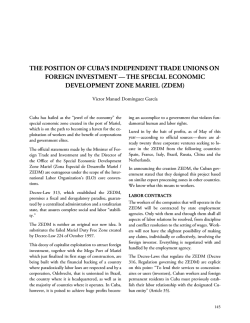# Jorge Perez-Lopez - Association for the Study of the Cuban Economy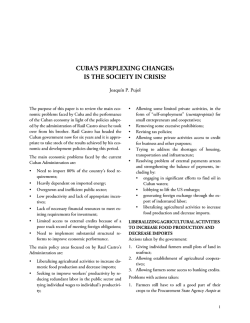# Jorge Perez-Lopez - Association for the Study of the Cuban Economy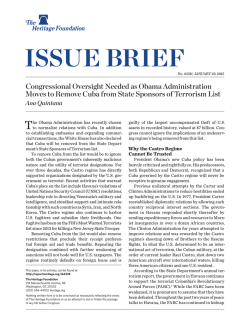# Obama Moves to Remove Cuba from State Sponsors of Terrorism List# Flyer con toda la informacion correcto San Francisco - CultuCuba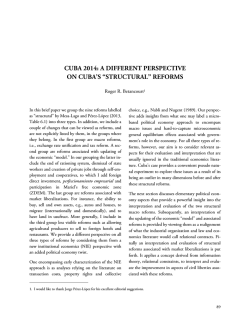# reforms - Association for the Study of the Cuban Economy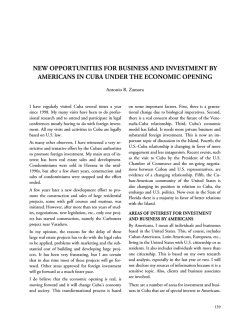# Jorge Perez-Lopez - Association for the Study of the Cuban Economy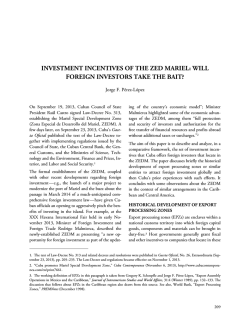# Jorge Perez-Lopez - Association for the Study of the Cuban Economy